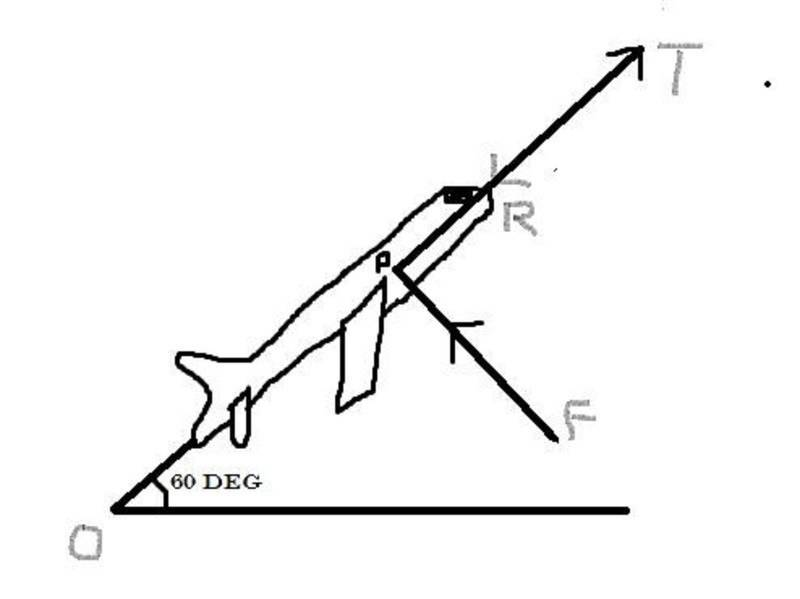# How to calculate K.E of this skater?

An ice skater spins about a vertical axis at an angular speed of 15 rad/sec when her arms are outstretched. She then quickly pulls her arms into her sides in a very small time interval so that the frictional forces due to ice are negligible. Her initial moment of inertia about the axis of rotation is 1.72 kg m2 and her final moment of inertia is 0.61 kg m2. What is the
change in her angular speed? What is the change in her kinetic energy? Explain this change
in kinetic energy.

## Answers and Replies

The angular momentum is conserved as there is no net external torque on the skater. Equate it for her final and initial states to find the new angular velocity.

Thank you pi-bond...yep i got the idea and ill pick up with this....can you kindly help me with this question too...
A bus is moving downhill at a slope of 5°. At the moment when the speed of the bus is
30 km/h, the driver spots a deer 30m ahead. He applies the brakes and comes to a stop. The deer is paralyzed by fear and does not move. Will the bus stop before reaching it or will it hit the deer? Do relevant calculations and draw appropriate force diagram. Take the coefficient of kinetic friction to be µk = 0.26.

I calculated the velocity of the bus when it reaches the deer using the formula, Work done = 1/2 mv1 square - 1/2 mv2 square....where work done is given by -µ . m . g . d....the velocity of the bus comes around 27 km/h when it reaches the deer...is my equation correct? if not kindly suggest the method of solving this question...

You also have to consider the potential energy which the bus has at it's top position; find the height between the two positions and then include the potential energy in your conservation equation.

Thank you again Pi-Bond...can you kindly clear another doubt of mine...sorry for bugging...here's the question:

A plane is flying with a constant speed along a straight line at an angle of 60° with the
horizontal. The weight W of the plane is 90, 000 N and its engine provides a thrust T of
120, 000 N in the direction of flight. Two additional forces are exerted on the plane: the lift force F perpendicular to the plane’s wings, and the force R due to air resistance opposite to the direction of motion. Determine F and R.

Can you kindly tell me what steps and formulae should be used to calculate F and R?

Can you draw a free body diagram showing all forces?O is the startin point. OT gives the direction and magnitude of Thrust. RP stands for the resistance of air ( drag?) . FP is the Force (Lift) that acts perpendicular to the wings. Am sure I've got one or two of these wrong. Kindly correct.

You forgot the weight, otherwise it is all right. Now resolve the forces along horizontal and vertical directions. Can you use the fact that the plane moves in a straight line with constant velocity to write an equality for the resolved forces?

horizontal forces are the resistance of air and the thrust acting opposite to each other.
Vertical forces are the Force of Lift and the weight of the plane acting opposite to each other. Bur how do i derive an equation based on this statement?

The forces due to thrust, air resistance and lift are not purely horizontal or vertical, as they are at an angle to the vertical and horizontal axes. You need to resolve them to these axes, and then use Newton's second law for horizontal and vertical forces.